Published

July 1, 2023

# Preparing a retention time data set for machine learning

``````# Skip if pygam already installed
! pip install pygam==0.8.0
! pip install tqdm==4.64.1``````
``````Requirement already satisfied: pygam in c:\users\robbin\anaconda3\envs\py310\lib\site-packages (0.8.0)
Requirement already satisfied: numpy in c:\users\robbin\anaconda3\envs\py310\lib\site-packages (from pygam) (1.23.3)
Requirement already satisfied: progressbar2 in c:\users\robbin\anaconda3\envs\py310\lib\site-packages (from pygam) (4.0.0)
Requirement already satisfied: scipy in c:\users\robbin\anaconda3\envs\py310\lib\site-packages (from pygam) (1.9.1)
Requirement already satisfied: future in c:\users\robbin\anaconda3\envs\py310\lib\site-packages (from pygam) (0.18.2)
Requirement already satisfied: python-utils>=3.0.0 in c:\users\robbin\anaconda3\envs\py310\lib\site-packages (from progressbar2->pygam) (3.3.3)
Requirement already satisfied: tqdm in c:\users\robbin\anaconda3\envs\py310\lib\site-packages (4.64.1)
Requirement already satisfied: colorama in c:\users\robbin\anaconda3\envs\py310\lib\site-packages (from tqdm) (0.4.5)``````
``````from collections import Counter
import os

from pygam import LinearGAM, s, f
from matplotlib import pyplot as plt
import pandas as pd
import numpy as np
from scipy.stats import pearsonr, spearmanr
from tqdm import tqdm

import warnings
warnings.filterwarnings("ignore")``````

In this tutorial you will learn how to go from MaxQuant evidence files to a data set that is ready for training a retention time prediction model. Retention time is the time it takes for an analyte travels through a column. The travel time depends on the interaction with the stationary phase (usually C18 for proteomics) and mobile phase. Where the mobile phase consists of solvents and changes in physicochemical properties over time with a predefined gradient. The stationary phase remains the same over time. This allows for peptides to elude at different time points, e.g., when it prefers to interact with the mobile phase at a certain percentage of the hydrophobic solvent.

The retention time between different runs can differ significantly and depending on the abundance of the precusor calling the elution apex can be difficult. This means we need to preprocess the data before it is used for machine learning.

## Reading and formatting input data

We will not need all the columns, define those that might be useful:

``````sel_columns = ['Raw file', 'Sequence', 'Modifications', 'Modified sequence',
'Retention time','Calibrated retention time', 'PEP']``````

Read the input files, here a csv. If you read the standard txt you need to modify the read_csv with:

`pd.read_csv("evid_files/PXD028248_evidence_selected_columns.csv",sep="\t",low_memory=False)`

Fill all the NA values with 0.0 and filter on only the most confident identifications (PEP <= 0.001).

``````evid_df = pd.read_csv("https://github.com/ProteomicsML/ProteomicsML/blob/main/datasets/retentiontime/PXD028248/PXD028248_evidence_selected_columns.zip?raw=true",compression="zip",low_memory=False)
evid_df.fillna(0.0,inplace=True)
evid_df = evid_df[evid_df["PEP"] <= 0.001][sel_columns]``````

The file in a pandas dataframe looks like this:

``evid_df``
Raw file Sequence Length Modifications Modified sequence Retention time Retention length Calibrated retention time Calibrated retention time start Calibrated retention time finish Retention time calibration Match time difference Intensity PEP
0 20191028_SJ_QEx_LC1200_4_Sommerfeld_OC218_ADP_... AAAAAAAAAAGAAGGR 16 Acetyl (Protein N-term) _(Acetyl (Protein N-term))AAAAAAAAAAGAAGGR_ 90.531 0.58251 90.531 90.202 90.784 0.000000e+00 0.0 40715000.0 9.822100e-15
1 20191126_SJ_QEx_LC1200_4_Sommerfeld_OC218_Tumo... AAAAAAAAAAGAAGGR 16 Acetyl (Protein N-term) _(Acetyl (Protein N-term))AAAAAAAAAAGAAGGR_ 97.132 0.51271 97.132 96.824 97.337 0.000000e+00 0.0 19359000.0 4.269700e-21
2 20191129_SJ_QEx_LC1200_4_Sommerfeld_OC193_Tumo... AAAAAAAAAAGAAGGR 16 Acetyl (Protein N-term) _(Acetyl (Protein N-term))AAAAAAAAAAGAAGGR_ 97.495 0.82283 97.495 96.848 97.671 0.000000e+00 0.0 173850000.0 1.198900e-42
3 20191129_SJ_QEx_LC1200_4_Sommerfeld_OC193_Tumo... AAAAAAAAAAGAAGGR 16 Acetyl (Protein N-term) _(Acetyl (Protein N-term))AAAAAAAAAAGAAGGR_ 96.580 0.46060 96.580 96.321 96.781 0.000000e+00 0.0 10126000.0 3.280800e-05
4 20191204_SJ_QEx_LC1200_4_Sommerfeld_OC217_Tumo... AAAAAAAAAAGAAGGR 16 Acetyl (Protein N-term) _(Acetyl (Protein N-term))AAAAAAAAAAGAAGGR_ 91.611 0.58345 91.611 91.341 91.924 0.000000e+00 0.0 16703000.0 4.950100e-17
... ... ... ... ... ... ... ... ... ... ... ... ... ... ...
1056802 20191204_SJ_QEx_LC1200_4_Sommerfeld_OC189_Tumo... YYYVCQYCPAGNWANR 16 Unmodified _YYYVCQYCPAGNWANR_ 93.503 0.45542 93.503 93.299 93.754 0.000000e+00 0.0 4054800.0 8.095500e-04
1056803 20191204_SJ_QEx_LC1200_4_Sommerfeld_OC196_Tumo... YYYVCQYCPAGNWANR 16 Unmodified _YYYVCQYCPAGNWANR_ 93.772 0.57550 93.772 93.492 94.067 0.000000e+00 0.0 13780000.0 2.618200e-04
1056804 20191204_SJ_QEx_LC1200_4_Sommerfeld_OC217_Tumo... YYYVCQYCPAGNWANR 16 Unmodified _YYYVCQYCPAGNWANR_ 93.183 0.60296 93.183 92.890 93.493 -1.421100e-14 0.0 9741300.0 1.367500e-06
1056805 20191210_SJ_QEx_LC1200_4_Sommerfeld_OC221_Tumo... YYYVCQYCPAGNWANR 16 Unmodified _YYYVCQYCPAGNWANR_ 95.546 0.50088 95.546 95.292 95.793 -1.421100e-14 0.0 7791200.0 1.803700e-11
1056806 20200229_SJ_QEx_LC1200_4_Sommerfeld_OC221_CAF_... YYYVCQYCPAGNWANR 16 Unmodified _YYYVCQYCPAGNWANR_ 69.370 0.21532 69.370 69.250 69.466 0.000000e+00 0.0 6157000.0 7.497600e-04

1056807 rows × 14 columns

As you can see in this example there are many of the same peptidoforms (minus charge) for the different runs. We will want to create a single value for each peptidoform per run in a matrix instead of a single peptidoform+run combo per row.

``````retention_dict = {}

# Group by the raw file
for gidx,g in evid_df.groupby("Raw file"):
# Group by peptidoform and take the mean for each group
retention_dict[gidx]  = g.groupby("Modified sequence").mean()["Calibrated retention time"].to_dict()

#Transform the dictionary in a df where each row is a peptidoform and each column a run
retention_df = pd.DataFrame(retention_dict)

retention_df``````
_(Acetyl (Protein N-term))ACGLVASNLNLKPGECLR_ 106.540 NaN NaN NaN NaN NaN NaN NaN NaN NaN ... NaN NaN 73.638 NaN NaN NaN 72.596 NaN NaN NaN
_(Acetyl (Protein N-term))AEEGIAAGGVM(Oxidation (M))DVNTALQEVLK_ 138.075 NaN NaN NaN NaN NaN NaN NaN NaN NaN ... NaN 121.063333 121.605 NaN NaN NaN NaN NaN NaN NaN
_(Acetyl (Protein N-term))AGWNAYIDNLM(Oxidation (M))ADGTCQDAAIVGYK_ 136.850 NaN NaN NaN NaN NaN NaN NaN NaN NaN ... NaN 107.397250 107.130 NaN NaN NaN NaN NaN NaN NaN
_(Acetyl (Protein N-term))SDAAVDTSSEITTK_ 62.757 NaN NaN NaN NaN NaN NaN NaN NaN NaN ... NaN 34.013000 NaN NaN NaN NaN NaN NaN NaN NaN
_AADDTWEPFASGK_ 90.319 89.749 NaN 88.939 NaN NaN NaN NaN NaN 105.24 ... NaN 55.282000 54.873 52.801 NaN NaN NaN NaN NaN NaN
... ... ... ... ... ... ... ... ... ... ... ... ... ... ... ... ... ... ... ... ... ...
_TALLTWTEPPVR_ NaN NaN NaN NaN NaN NaN NaN NaN NaN NaN ... NaN NaN NaN NaN NaN NaN NaN NaN NaN 76.442
_TQFNNNEYSQDLDAYNTKDK_ NaN NaN NaN NaN NaN NaN NaN NaN NaN NaN ... NaN NaN NaN NaN NaN NaN NaN NaN NaN 45.327
_VATGTDLLSGTR_ NaN NaN NaN NaN NaN NaN NaN NaN NaN NaN ... NaN NaN NaN NaN NaN NaN NaN NaN NaN 36.155
_VNWMPPPSR_ NaN NaN NaN NaN NaN NaN NaN NaN NaN NaN ... NaN NaN NaN NaN NaN NaN NaN NaN NaN 40.657
_VTDIDSDDHQVM(Oxidation (M))YIM(Oxidation (M))K_ NaN NaN NaN NaN NaN NaN NaN NaN NaN NaN ... NaN NaN NaN NaN NaN NaN NaN NaN NaN 32.140

49983 rows × 441 columns

We can than have a look at the absence of each peptidoform in all runs (value = absence in that many runs):

``````prevelence_peptides = retention_df.isna().sum(axis=1)
print(prevelence_peptides)``````
``````_(Acetyl (Protein N-term))ACGLVASNLNLKPGECLR_                          385
_(Acetyl (Protein N-term))AEEGIAAGGVM(Oxidation (M))DVNTALQEVLK_       408
_(Acetyl (Protein N-term))SDAAVDTSSEITTK_                              430
...
_TALLTWTEPPVR_                                                         440
_TQFNNNEYSQDLDAYNTKDK_                                                 440
_VATGTDLLSGTR_                                                         440
_VNWMPPPSR_                                                            440
_VTDIDSDDHQVM(Oxidation (M))YIM(Oxidation (M))K_                       440
Length: 49983, dtype: int64``````

We can penalize the absence the absence of highly abundant peptidoforms per run (lower = more abundant peptidoforms present) by taking the dot product of presence/absence in the matrix and the above absence scores:

``````score_per_run = retention_df.isna().astype(int).T.dot(prevelence_peptides)
score_per_run.sort_values(ascending=True)``````
``````20200319_SJ_QEx_LC1200_4_Sommerfeld_OC186_HPMC_50Asc_498     18417985
20200319_SJ_QEx_LC1200_4_Sommerfeld_OC186_HPMC_50Asc_499     18633580
20200319_SJ_QEx_LC1200_4_Sommerfeld_OC186_HPMC_50Asc_502     18802678
20200319_SJ_QEx_LC1200_4_Sommerfeld_OC186_HPMC_50Asc_501     18807279
20191129_SJ_QEx_LC1200_4_Sommerfeld_OC193_Tumor_50Asc_138    18877939
...
20191028_SJ_QEx_LC1200_4_Sommerfeld_0Wert_HPMC_FCS_85        21261614
20191121_SJ_QEx_LC1200_4_Sommerfeld_0Wert_CAF_FCS_100        21262661
20191028_SJ_QEx_LC1200_4_Sommerfeld_0Wert_HPMC_FCS_83        21263993
20191028_SJ_QEx_LC1200_4_Sommerfeld_0Wert_HPMC_FCS_82        21265683
20191028_SJ_QEx_LC1200_4_Sommerfeld_0Wert_HPMC_FCS_87        21268762
Length: 441, dtype: int64``````

We will use a single run to align all the first experiments against, this is the one with the lowest penalty score:

``run_highest_overlap_score = score_per_run.sort_values(ascending=True).index``

## Retention time alignment between runs

The first step after reading and loading the data is to align retention times between runs. Here we will use splines in a GAM for that. The algorithm below follows these steps:

1. Iterate over all runs, sorted by the earlier defined penalty score
2. Obtain the overlapping peptidoforms between runs
3. If there are less than 20 peptidoforms skip that run
4. Divide the overlapping peptides into equidistant bins and enforce a percentage of the bins to be filled with a least one peptidoform (now 200 bins and 75 % occupancy). If requirements are not met skip that run.
5. Fit the GAM with splines between the reference set and the selected run
6. Calculate the error between aligned and values in the reference set. If selected it will run a second stage of the GAM filtering out any data points that were selected to have an error that is too high
7. Assign aligned values to a new matrix
8. Change the reference dataset to be the median of all aligned runs and the initial reference run

In the next code block we will define two kinds of plots, first a performance scatter plot. Here we plot the retention time of the selected set against the reference set; before and after alignment. Next is the residual plot that subtracts the diagonal from the performance scatter plot and essentially shows the errors before and after alignment. The residual plot is generated for both the first and second stage GAM.

``````def plot_performance(retention_df,run_highest_overlap_score,align_name,non_na_sel):
plt.scatter(
retention_df[run_highest_overlap_score][non_na_sel],
retention_df[align_name][non_na_sel],
alpha=0.05,
s=10,
label="Reference+selected set unaligned"
)

plt.scatter(
retention_df[run_highest_overlap_score][non_na_sel],
gam_model_cv.predict(retention_df[align_name][non_na_sel]),
alpha=0.05,
s=10,
label="Reference+selected set aligned"
)
plt.plot(
[
min(retention_df[run_highest_overlap_score][non_na_sel]),
max(retention_df[run_highest_overlap_score][non_na_sel])

],
[
min(retention_df[run_highest_overlap_score][non_na_sel]),
max(retention_df[run_highest_overlap_score][non_na_sel])

],
c="black",
linestyle="--",
linewidth=1.0
)
plt.xlabel("Retention time reference set")
plt.ylabel("Retention time selected set")
leg = plt.legend()
for lh in leg.legendHandles:
lh.set_alpha(1)

plt.show()

def plot_residual(run_highest_overlap_score,align_name,non_na_sel,title="Residual plot"):
plt.scatter(
retention_df[run_highest_overlap_score][non_na_sel],
retention_df[align_name][non_na_sel]-retention_df[run_highest_overlap_score][non_na_sel],
alpha=0.05,
s=10
)

plt.scatter(
retention_df[run_highest_overlap_score][non_na_sel],
gam_model_cv.predict(retention_df[align_name][non_na_sel])-retention_df[run_highest_overlap_score][non_na_sel],
alpha=0.05,
s=10
)

plt.title(title)

plt.axhline(
y = 0.0,
color = "black",
linewidth=1.0,
linestyle = "--"
)

plt.ylabel("Residual")
plt.xlabel("Retention time reference")

plt.show()``````
``````#constraints = "monotonic_inc"
constraints = "none"

# Align parameters
perform_second_stage_robust = True
error_filter_perc = 0.005
num_splines = 150
min_coverage = 0.75
coverage_div = 200
plot_res_every_n = 100
min_overlap = 20

run_highest_overlap_score = score_per_run.sort_values(ascending=True).index

unique_peptides = []
unique_peptides.extend(list(retention_df[retention_df[run_highest_overlap_score].notna()].index))

retention_df_aligned = retention_df.copy()

keep_cols = [run_highest_overlap_score]

error_filter_perc_threshold = max(retention_df[run_highest_overlap_score])*error_filter_perc

# Iterate over runs sorted by a penalty score
# For version 3.8 or later uncomment the for loop below and comment the other for loop; also uncomment the line after update progressbar
#for idx,align_name in (pbar := tqdm(enumerate(score_per_run.sort_values(ascending=True)[1:].index))):
for idx,align_name in tqdm(enumerate(score_per_run.sort_values(ascending=True)[1:].index)):
# Update progressbar
#pbar.set_description(f"Processing {align_name}")

# Check overlap between peptidoforms
non_na_sel = (retention_df[align_name].notna()) & (retention_df[run_highest_overlap_score].notna())

# Continue if insufficient overlapping peptides
if len(retention_df[run_highest_overlap_score][non_na_sel].index) < min_overlap:
continue

# Check spread of overlapping peptidoforms, continue if not sufficient
if (len(set(pd.cut(retention_df[align_name][non_na_sel], coverage_div, include_lowest = True))) / coverage_div) < min_coverage:
continue

# Fit the GAM
gam_model_cv = LinearGAM(s(0, n_splines=num_splines), constraints=constraints, verbose=True).fit(
retention_df[align_name][non_na_sel],
retention_df[run_highest_overlap_score][non_na_sel])

# Plot results alignment
if idx % plot_res_every_n == 0 or idx == 0:
plot_performance(
retention_df,
run_highest_overlap_score,
align_name,
non_na_sel
)
plot_residual(
run_highest_overlap_score,
align_name,
non_na_sel
)

# Calculate errors and create filter that can be used in the second stage
errors = abs(gam_model_cv.predict(retention_df[align_name][non_na_sel])-retention_df[run_highest_overlap_score][non_na_sel])
error_filter = errors < error_filter_perc_threshold

# Perform a second stage GAM removing high error from previous fit
if perform_second_stage_robust:
gam_model_cv = LinearGAM(s(0, n_splines=num_splines), constraints=constraints, verbose=True).fit(
retention_df[align_name][non_na_sel][error_filter],
retention_df[run_highest_overlap_score][non_na_sel][error_filter])

if idx % plot_res_every_n == 0  or idx == 0:
plot_residual(
run_highest_overlap_score,
align_name,
non_na_sel,
title="Residual plot second stage GAM"
)

# Write alignment to new matrix
retention_df_aligned.loc[retention_df[align_name].notna(),align_name] = gam_model_cv.predict(retention_df.loc[retention_df[align_name].notna(),align_name])

unique_peptides.extend(list(retention_df[retention_df[align_name].notna()].index))

keep_cols.append(align_name)

# Create reference set based on aligned retention times
retention_df["median_aligned"] = retention_df_aligned[keep_cols].median(axis=1)
run_highest_overlap_score = "median_aligned"``````
``Processing 20200319_SJ_QEx_LC1200_4_Sommerfeld_OC186_HPMC_50Asc_499: : 0it [00:00, ?it/s]Processing 20200109_SJ_QEx_LC1200_4_Sommerfeld_OC221_Tumor_5FCS_340: : 100it [01:23,  1.29it/s]Processing 20200109_SJ_QEx_LC1200_4_Sommerfeld_OC217_Tumor_5FCS_318: : 200it [02:42,  1.42it/s] Processing 20191210_SJ_QEx_LC1200_4_Sommerfeld_OC195_Tumor_50Asc_280: : 300it [03:54,  1.37it/s]Processing 20191028_SJ_QEx_LC1200_4_Sommerfeld_0Wert_HPMC_FCS_87: : 440it [04:40,  1.57it/s]    ``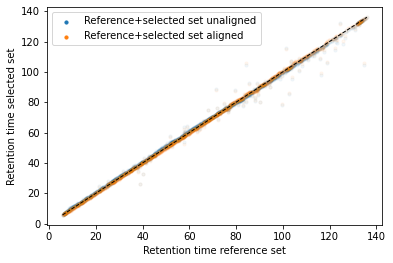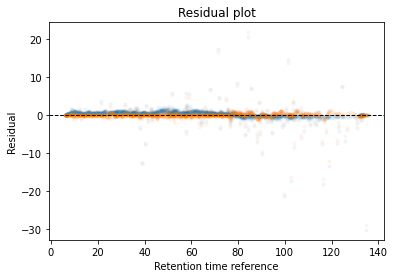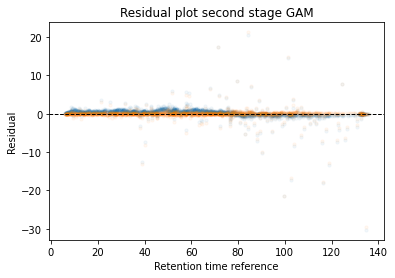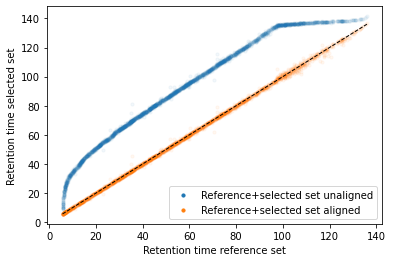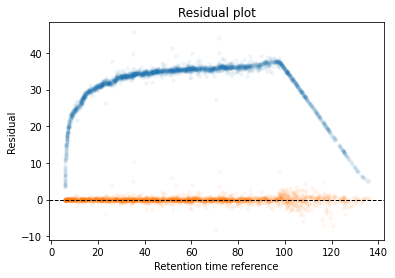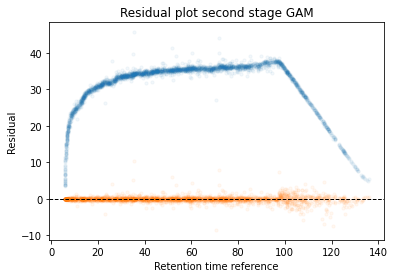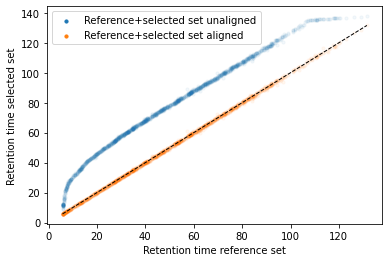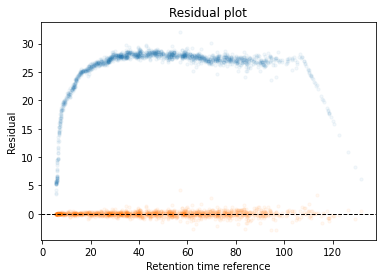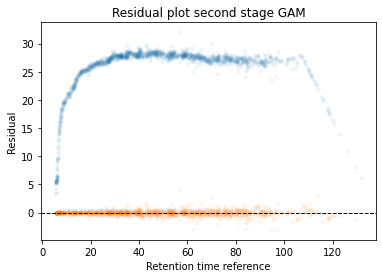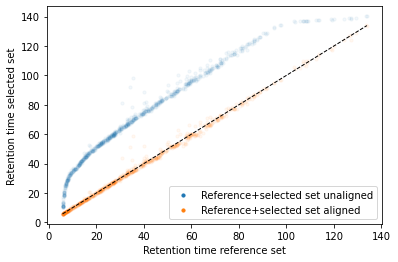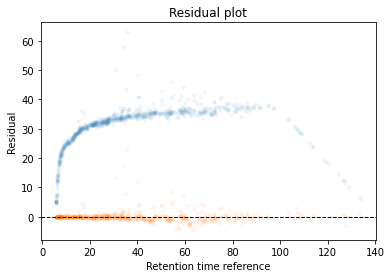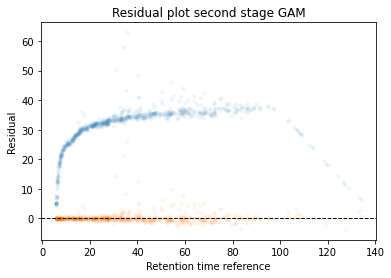The data points acquired looks as the following vector:

``retention_df_aligned[keep_cols].median(axis=1)``
``````_(Acetyl (Protein N-term))ACGLVASNLNLKPGECLR_                           70.639004
_(Acetyl (Protein N-term))AEEGIAAGGVM(Oxidation (M))DVNTALQEVLK_       118.580954
_(Acetyl (Protein N-term))SDAAVDTSSEITTK_                               28.451475
...
_TALLTWTEPPVR_                                                          70.065188
_TQFNNNEYSQDLDAYNTKDK_                                                  39.432309
_VATGTDLLSGTR_                                                          30.655512
_VNWMPPPSR_                                                             35.224075
_VTDIDSDDHQVM(Oxidation (M))YIM(Oxidation (M))K_                        27.263047
Length: 49983, dtype: float64``````

If we look at the standard deviation we can see that this is still relatively large for some peptidoforms:

``````plt.hist(retention_df_aligned[keep_cols].std(axis=1),bins=500)
plt.xlabel("Standard deviation retention time")
plt.show()

plt.hist(retention_df_aligned[keep_cols].std(axis=1),bins=500)
plt.xlim(0,7.5)
plt.xlabel("Standard deviation retention time (zoomed)")
plt.show()``````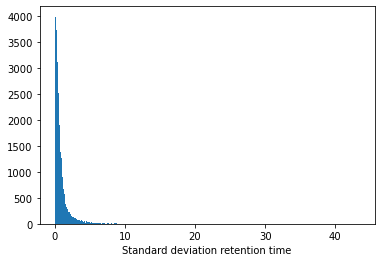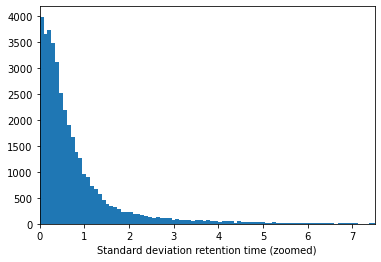In addition to the std there is another factor that can play a big role, the amount of times a peptidoform was observed:

``````plt.hist(retention_df_aligned[keep_cols].notna().sum(axis=1),bins=100)
plt.xlabel("Count peptidoforms across runs")
plt.show()

plt.hist(retention_df_aligned[keep_cols].notna().sum(axis=1),bins=100)
plt.xlabel("Count peptidoforms across runs (zoomed)")
plt.xlim(0,20)
plt.show()``````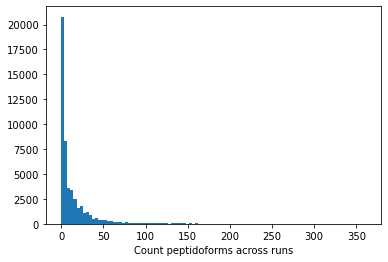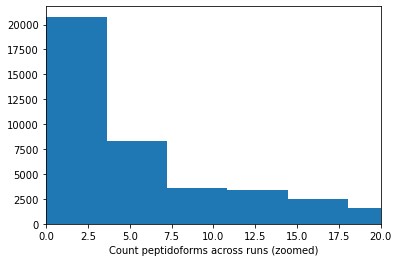If we plot both values against each other we get the following plot (the lines indicate possible thresholds):

``````plt.scatter(
retention_df_aligned[keep_cols].notna().sum(axis=1),
retention_df_aligned[keep_cols].std(axis=1),
s=5,
alpha=0.1
)

plt.ylabel("Standard deviation retention time")
plt.xlabel("Count peptidoforms across runs")

plt.axhline(
y = 2.0,
color = "black",
linewidth=1.0,
linestyle = "--"
)

plt.axvline(
x = 5.0,
color = "black",
linewidth=1.0,
linestyle = "--"
)

plt.show()``````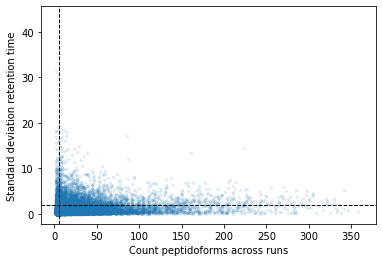If we set a threshold for a minimum of 5 observations and a maximum standard deviation of 2 we get the following final data set. Here we take the median for each peptidoform across all runs:

``````min_observations = 5
max_std = 2.0

observation_filter = retention_df_aligned[keep_cols].notna().sum(axis=1) > min_observations
std_filter = retention_df_aligned[keep_cols].std(axis=1) < max_std

retention_df_aligned[keep_cols][(observation_filter) & (std_filter)].median(axis=1)``````
``````_(Acetyl (Protein N-term))SDAAVDTSSEITTK_     28.451475